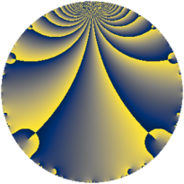# Properties

 Label 2898.2.a.zLevel $2898$ Weight $2$ Character orbit 2898.a Self dual yes Analytic conductor $23.141$ Analytic rank $1$ Dimension $2$ CM no Inner twists $1$

# Related objects

## Newspace parameters

 Level: $$N$$ $$=$$ $$2898 = 2 \cdot 3^{2} \cdot 7 \cdot 23$$ Weight: $$k$$ $$=$$ $$2$$ Character orbit: $$[\chi]$$ $$=$$ 2898.a (trivial)

## Newform invariants

 Self dual: yes Analytic conductor: $$23.1406465058$$ Analytic rank: $$1$$ Dimension: $$2$$ Coefficient field: $$\Q(\sqrt{2})$$ Defining polynomial: $$x^{2} - 2$$ Coefficient ring: $$\Z[a_1, \ldots, a_{5}]$$ Coefficient ring index: $$1$$ Twist minimal: yes Fricke sign: $$1$$ Sato-Tate group: $\mathrm{SU}(2)$

## $q$-expansion

Coefficients of the $$q$$-expansion are expressed in terms of $$\beta = \sqrt{2}$$. We also show the integral $$q$$-expansion of the trace form.

 $$f(q)$$ $$=$$ $$q + q^{2} + q^{4} + ( -2 + \beta ) q^{5} - q^{7} + q^{8} +O(q^{10})$$ $$q + q^{2} + q^{4} + ( -2 + \beta ) q^{5} - q^{7} + q^{8} + ( -2 + \beta ) q^{10} -3 \beta q^{11} + ( -2 - 2 \beta ) q^{13} - q^{14} + q^{16} + ( 2 + 2 \beta ) q^{17} + 5 \beta q^{19} + ( -2 + \beta ) q^{20} -3 \beta q^{22} + q^{23} + ( 1 - 4 \beta ) q^{25} + ( -2 - 2 \beta ) q^{26} - q^{28} + 2 q^{29} + ( -2 - 2 \beta ) q^{31} + q^{32} + ( 2 + 2 \beta ) q^{34} + ( 2 - \beta ) q^{35} + ( -6 + 3 \beta ) q^{37} + 5 \beta q^{38} + ( -2 + \beta ) q^{40} -2 q^{41} + ( -8 - \beta ) q^{43} -3 \beta q^{44} + q^{46} + ( -6 - 2 \beta ) q^{47} + q^{49} + ( 1 - 4 \beta ) q^{50} + ( -2 - 2 \beta ) q^{52} + ( -6 - 3 \beta ) q^{53} + ( -6 + 6 \beta ) q^{55} - q^{56} + 2 q^{58} -2 \beta q^{59} + ( -10 - 3 \beta ) q^{61} + ( -2 - 2 \beta ) q^{62} + q^{64} + 2 \beta q^{65} + ( -8 - 3 \beta ) q^{67} + ( 2 + 2 \beta ) q^{68} + ( 2 - \beta ) q^{70} + ( 10 - 2 \beta ) q^{71} -12 q^{73} + ( -6 + 3 \beta ) q^{74} + 5 \beta q^{76} + 3 \beta q^{77} + 8 \beta q^{79} + ( -2 + \beta ) q^{80} -2 q^{82} + ( -8 - \beta ) q^{83} -2 \beta q^{85} + ( -8 - \beta ) q^{86} -3 \beta q^{88} + ( 2 + 4 \beta ) q^{89} + ( 2 + 2 \beta ) q^{91} + q^{92} + ( -6 - 2 \beta ) q^{94} + ( 10 - 10 \beta ) q^{95} + ( -6 - 2 \beta ) q^{97} + q^{98} +O(q^{100})$$ $$\operatorname{Tr}(f)(q)$$ $$=$$ $$2q + 2q^{2} + 2q^{4} - 4q^{5} - 2q^{7} + 2q^{8} + O(q^{10})$$ $$2q + 2q^{2} + 2q^{4} - 4q^{5} - 2q^{7} + 2q^{8} - 4q^{10} - 4q^{13} - 2q^{14} + 2q^{16} + 4q^{17} - 4q^{20} + 2q^{23} + 2q^{25} - 4q^{26} - 2q^{28} + 4q^{29} - 4q^{31} + 2q^{32} + 4q^{34} + 4q^{35} - 12q^{37} - 4q^{40} - 4q^{41} - 16q^{43} + 2q^{46} - 12q^{47} + 2q^{49} + 2q^{50} - 4q^{52} - 12q^{53} - 12q^{55} - 2q^{56} + 4q^{58} - 20q^{61} - 4q^{62} + 2q^{64} - 16q^{67} + 4q^{68} + 4q^{70} + 20q^{71} - 24q^{73} - 12q^{74} - 4q^{80} - 4q^{82} - 16q^{83} - 16q^{86} + 4q^{89} + 4q^{91} + 2q^{92} - 12q^{94} + 20q^{95} - 12q^{97} + 2q^{98} + O(q^{100})$$

## Embeddings

For each embedding $$\iota_m$$ of the coefficient field, the values $$\iota_m(a_n)$$ are shown below.

For more information on an embedded modular form you can click on its label.

Label $$\iota_m(\nu)$$ $$a_{2}$$ $$a_{3}$$ $$a_{4}$$ $$a_{5}$$ $$a_{6}$$ $$a_{7}$$ $$a_{8}$$ $$a_{9}$$ $$a_{10}$$
1.1
 −1.41421 1.41421
1.00000 0 1.00000 −3.41421 0 −1.00000 1.00000 0 −3.41421
1.2 1.00000 0 1.00000 −0.585786 0 −1.00000 1.00000 0 −0.585786
 $$n$$: e.g. 2-40 or 990-1000 Significant digits: Format: Complex embeddings Normalized embeddings Satake parameters Satake angles

## Atkin-Lehner signs

$$p$$ Sign
$$2$$ $$-1$$
$$3$$ $$1$$
$$7$$ $$1$$
$$23$$ $$-1$$

## Inner twists

This newform does not admit any (nontrivial) inner twists.

## Twists

By twisting character orbit
Char Parity Ord Mult Type Twist Min Dim
1.a even 1 1 trivial 2898.2.a.z yes 2
3.b odd 2 1 2898.2.a.y 2

By twisted newform orbit
Twist Min Dim Char Parity Ord Mult Type
2898.2.a.y 2 3.b odd 2 1
2898.2.a.z yes 2 1.a even 1 1 trivial

## Hecke kernels

This newform subspace can be constructed as the intersection of the kernels of the following linear operators acting on $$S_{2}^{\mathrm{new}}(\Gamma_0(2898))$$:

 $$T_{5}^{2} + 4 T_{5} + 2$$ $$T_{11}^{2} - 18$$ $$T_{13}^{2} + 4 T_{13} - 4$$

## Hecke characteristic polynomials

$p$ $F_p(T)$
$2$ $$( -1 + T )^{2}$$
$3$ $$T^{2}$$
$5$ $$2 + 4 T + T^{2}$$
$7$ $$( 1 + T )^{2}$$
$11$ $$-18 + T^{2}$$
$13$ $$-4 + 4 T + T^{2}$$
$17$ $$-4 - 4 T + T^{2}$$
$19$ $$-50 + T^{2}$$
$23$ $$( -1 + T )^{2}$$
$29$ $$( -2 + T )^{2}$$
$31$ $$-4 + 4 T + T^{2}$$
$37$ $$18 + 12 T + T^{2}$$
$41$ $$( 2 + T )^{2}$$
$43$ $$62 + 16 T + T^{2}$$
$47$ $$28 + 12 T + T^{2}$$
$53$ $$18 + 12 T + T^{2}$$
$59$ $$-8 + T^{2}$$
$61$ $$82 + 20 T + T^{2}$$
$67$ $$46 + 16 T + T^{2}$$
$71$ $$92 - 20 T + T^{2}$$
$73$ $$( 12 + T )^{2}$$
$79$ $$-128 + T^{2}$$
$83$ $$62 + 16 T + T^{2}$$
$89$ $$-28 - 4 T + T^{2}$$
$97$ $$28 + 12 T + T^{2}$$